# COP 4710 Database Systems Spring 2004 Day 11

• Slides: 30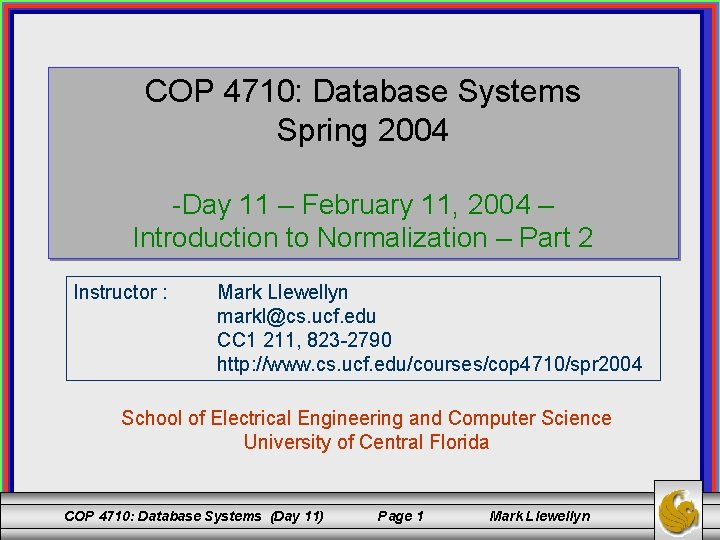COP 4710: Database Systems Spring 2004 -Day 11 – February 11, 2004 – Introduction to Normalization – Part 2 Instructor : Mark Llewellyn [email protected] ucf. edu CC 1 211, 823 -2790 http: //www. cs. ucf. edu/courses/cop 4710/spr 2004 School of Electrical Engineering and Computer Science University of Central Florida COP 4710: Database Systems (Day 11) Page 1 Mark LlewellynThird Normal Form (3 NF) • Third Normal Form (3 NF) is based on the concept of a transitive dependency. • Given a relation scheme R with a set of functional dependencies F and subset X R and an attribute A R. A is said to be transitively dependent on X if there exists Y R with X Y, Y X X and Y A and A X Y. • An alternative definition for a transitive dependency is: a functional dependency X Y in a relation scheme R is a transitive dependency if there is a set of attributes Z R where Z is not a subset of any key of R and yet both X Z and Z Y hold in F. COP 4710: Database Systems (Day 11) Page 2 Mark Llewellyn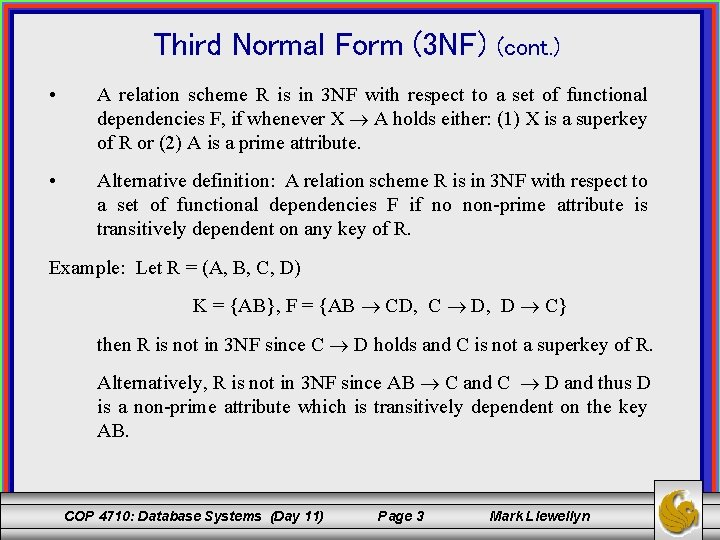Third Normal Form (3 NF) (cont. ) • A relation scheme R is in 3 NF with respect to a set of functional dependencies F, if whenever X A holds either: (1) X is a superkey of R or (2) A is a prime attribute. • Alternative definition: A relation scheme R is in 3 NF with respect to a set of functional dependencies F if no non-prime attribute is transitively dependent on any key of R. Example: Let R = (A, B, C, D) K = {AB}, F = {AB CD, C D, D C} then R is not in 3 NF since C D holds and C is not a superkey of R. Alternatively, R is not in 3 NF since AB C and C D and thus D is a non-prime attribute which is transitively dependent on the key AB. COP 4710: Database Systems (Day 11) Page 3 Mark Llewellyn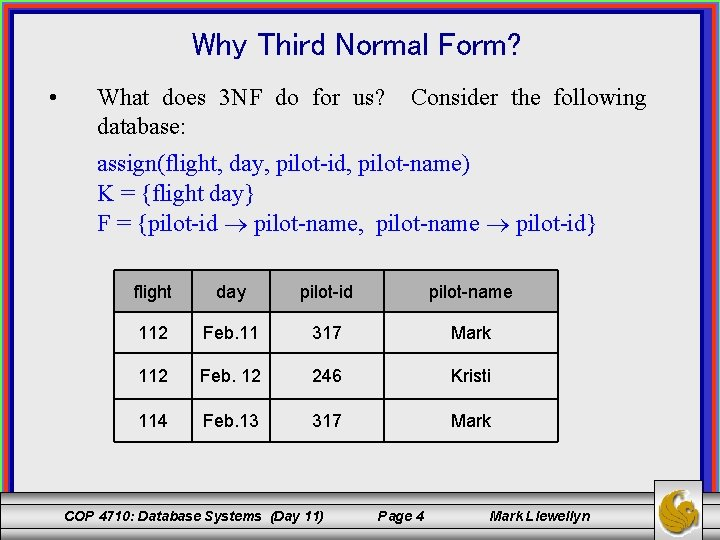Why Third Normal Form? • What does 3 NF do for us? database: Consider the following assign(flight, day, pilot-id, pilot-name) K = {flight day} F = {pilot-id pilot-name, pilot-name pilot-id} flight day pilot-id pilot-name 112 Feb. 11 317 Mark 112 Feb. 12 246 Kristi 114 Feb. 13 317 Mark COP 4710: Database Systems (Day 11) Page 4 Mark Llewellyn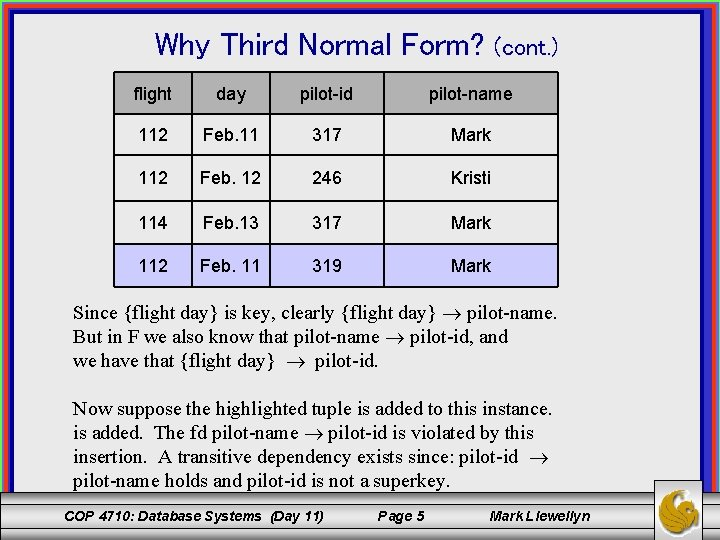Why Third Normal Form? (cont. ) flight day pilot-id pilot-name 112 Feb. 11 317 Mark 112 Feb. 12 246 Kristi 114 Feb. 13 317 Mark 112 Feb. 11 319 Mark Since {flight day} is key, clearly {flight day} pilot-name. But in F we also know that pilot-name pilot-id, and we have that {flight day} pilot-id. Now suppose the highlighted tuple is added to this instance. is added. The fd pilot-name pilot-id is violated by this insertion. A transitive dependency exists since: pilot-id pilot-name holds and pilot-id is not a superkey. COP 4710: Database Systems (Day 11) Page 5 Mark LlewellynBoyce-Codd Normal Form (BCNF) • Boyce-Codd Normal Form (BCNF) is a more stringent form of 3 NF. • A relation scheme R is in Boyce-Codd Normal Form with respect to a set of functional dependencies F if whenever X A hold and A ⊈ X, then X is a superkey of R. Example: Let R = (A, B, C) F = {AB C, C A} K= {AB} R is not in BCNF since C A holds and C is not a superkey of R. COP 4710: Database Systems (Day 11) Page 6 Mark Llewellyn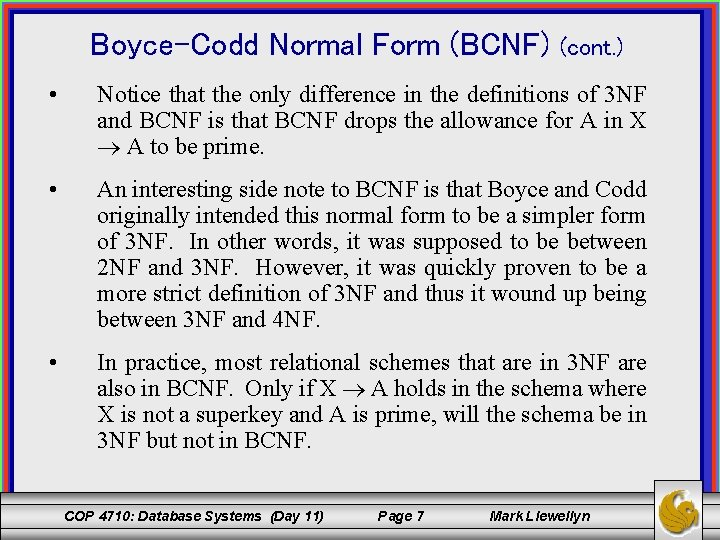Boyce-Codd Normal Form (BCNF) (cont. ) • Notice that the only difference in the definitions of 3 NF and BCNF is that BCNF drops the allowance for A in X A to be prime. • An interesting side note to BCNF is that Boyce and Codd originally intended this normal form to be a simpler form of 3 NF. In other words, it was supposed to be between 2 NF and 3 NF. However, it was quickly proven to be a more strict definition of 3 NF and thus it wound up being between 3 NF and 4 NF. • In practice, most relational schemes that are in 3 NF are also in BCNF. Only if X A holds in the schema where X is not a superkey and A is prime, will the schema be in 3 NF but not in BCNF. COP 4710: Database Systems (Day 11) Page 7 Mark LlewellynMoving Towards Relational Decomposition • The basic goal of relational database design should be to ensure that every relation in the database is either in 3 NF or BCNF. • 1 NF and 2 NF do not remove a sufficient number of the update anomalies to make a significant difference, whereas 3 NF and BCNF eliminate most of the update anomalies. • As we’ve mentioned before, in addition to ensuring the relation schemas are in either 3 NF or BCNF, the designer must also ensure that the decomposition of the original database schema into the 3 NF or BCNF schemas guarantees that the decomposition have (1) the lossless join property (also called a non-additive join property) and (2) the functional dependencies are preserved across the decomposition. COP 4710: Database Systems (Day 11) Page 8 Mark LlewellynMoving Towards Relational Decomposition (cont. ) • There are decomposition algorithms that will guarantee a 3 NF decomposition which ensures both the lossless join property and preservation of the functional dependencies. • However, there is no algorithm which will guarantee a BCNF decomposition which ensures both the lossless join property and preserves the functional dependencies. There is an algorithm that will guarantee BCNF and the lossless join property, but this algorithm cannot guarantee that the dependencies will be preserved. • It is for this reason that many times, 3 NF is as strong a normal form as will be possible for a certain set of schemas, since an attempt to force BCNF may result in the non-preservation of the dependencies. • In the next few pages we’ll look at these two properties more closely. COP 4710: Database Systems (Day 11) Page 9 Mark LlewellynPreservation of the Functional Dependencies • Whenever an update is made to the database, the DBMS must be able to verify that the update will not result in an illegal instance with respect to the functional dependencies in F+. • To check updates in an efficient manner the database must be designed with a set of schemas which allows for this verification to occur without necessitating join operations. • If an fd is “lost”, the only way to enforce the constraint would be to effect a join of two or more relations in the decomposition to get a “relation” that includes all of the determinant and consequent attributes of the lost fd into a single table, then verify that the dependency still holds after the update occurs. Obviously, this requires too much effort to be practical or efficient. COP 4710: Database Systems (Day 11) Page 10 Mark Llewellyn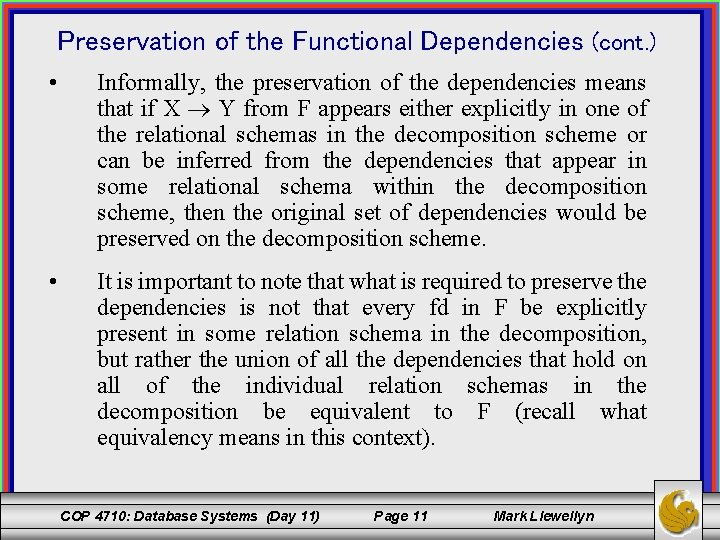Preservation of the Functional Dependencies (cont. ) • Informally, the preservation of the dependencies means that if X Y from F appears either explicitly in one of the relational schemas in the decomposition scheme or can be inferred from the dependencies that appear in some relational schema within the decomposition scheme, then the original set of dependencies would be preserved on the decomposition scheme. • It is important to note that what is required to preserve the dependencies is not that every fd in F be explicitly present in some relation schema in the decomposition, but rather the union of all the dependencies that hold on all of the individual relation schemas in the decomposition be equivalent to F (recall what equivalency means in this context). COP 4710: Database Systems (Day 11) Page 11 Mark LlewellynPreservation of the Functional Dependencies (cont. ) • The projection of a set of functional dependencies onto a set of attributes Z, denoted F[Z] (also sometime as Z(F)), is the set of functional dependencies X Y in F+ such that X Y Z. • A decomposition scheme = {R 1, R 2, …, Rm} is dependency preserving with respect to a set of fds F if the union of the projection of F onto each Ri (1 i m) in is equivalent to F. (F[R 1] F[R 2] … F[Rm])+ = F+ COP 4710: Database Systems (Day 11) Page 12 Mark Llewellyn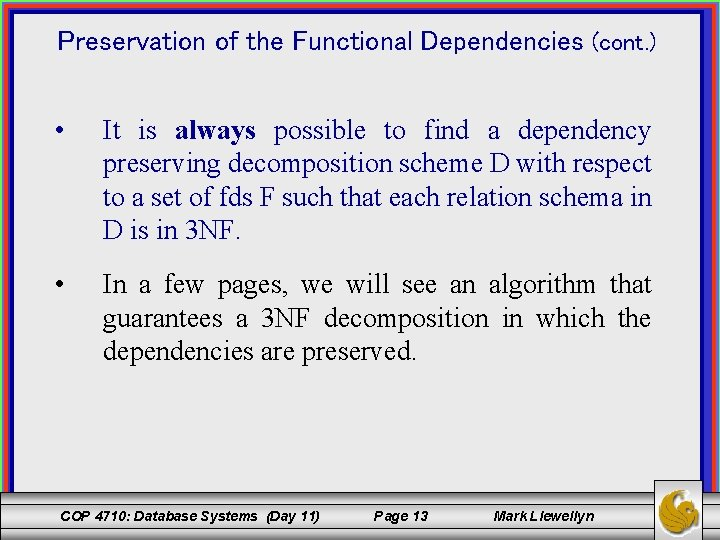Preservation of the Functional Dependencies (cont. ) • It is always possible to find a dependency preserving decomposition scheme D with respect to a set of fds F such that each relation schema in D is in 3 NF. • In a few pages, we will see an algorithm that guarantees a 3 NF decomposition in which the dependencies are preserved. COP 4710: Database Systems (Day 11) Page 13 Mark Llewellyn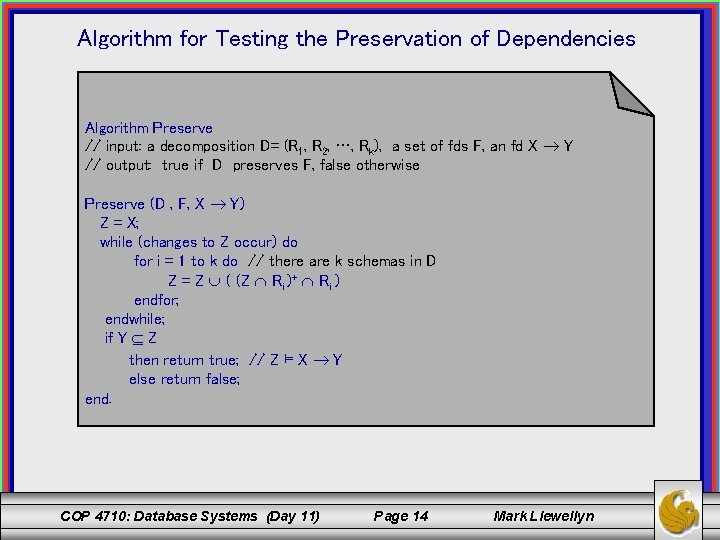Algorithm for Testing the Preservation of Dependencies Algorithm Preserve // input: a decomposition D= (R 1, R 2, …, Rk), a set of fds F, an fd X Y // output: true if D preserves F, false otherwise Preserve (D , F, X Y) Z = X; while (changes to Z occur) do for i = 1 to k do // there are k schemas in D Z = Z ( (Z Ri )+ Ri ) endfor; endwhile; if Y Z then return true; // Z ⊨ X Y else return false; end. COP 4710: Database Systems (Day 11) Page 14 Mark LlewellynHow Algorithm Preserves Works • The set Z which is computed is basically the following: • Note that G is not actually computed but merely tested to see if G covers F. To test if G covers F we need to consider each fd X Y in F and determine if contains Y. • Thus, the technique is to compute without having G available by repeatedly considering the effect of closing F with respect to the projections of F onto the various Ri. COP 4710: Database Systems (Day 11) Page 15 Mark LlewellynA Hugmongously Big Example Let R = (A, B, C, D) F = {A B, B C, C D, D A} D = {(AB), (BC), (CD)} G = F[AB] F[BC] F[CD] Z = Z ((Z Ri)+ Ri) Test for each fd in F. Test for A B Z = A, = {A} ((A AB)+ AB) = {A} ((A)+ AB) = {A} (ABCD AB) = {A} {AB} = {AB} COP 4710: Database Systems (Day 11) Page 16 Mark Llewellyn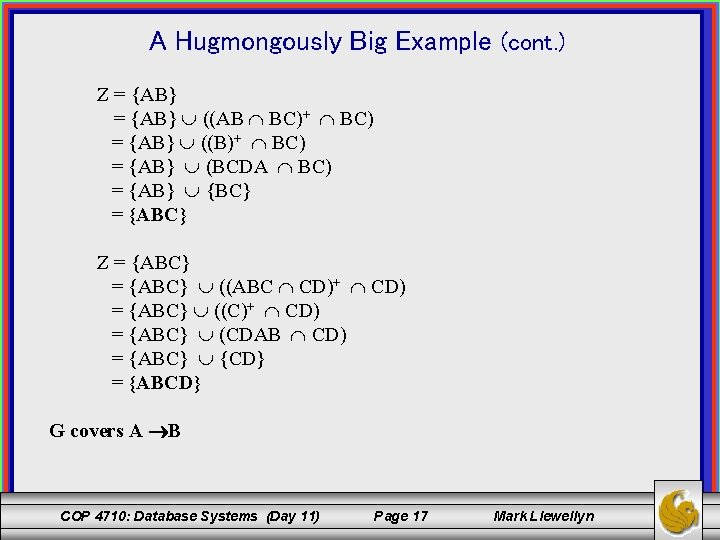A Hugmongously Big Example (cont. ) Z = {AB} ((AB BC)+ BC) = {AB} ((B)+ BC) = {AB} (BCDA BC) = {AB} {BC} = {ABC} Z = {ABC} ((ABC CD)+ CD) = {ABC} ((C)+ CD) = {ABC} (CDAB CD) = {ABC} {CD} = {ABCD} G covers A B COP 4710: Database Systems (Day 11) Page 17 Mark Llewellyn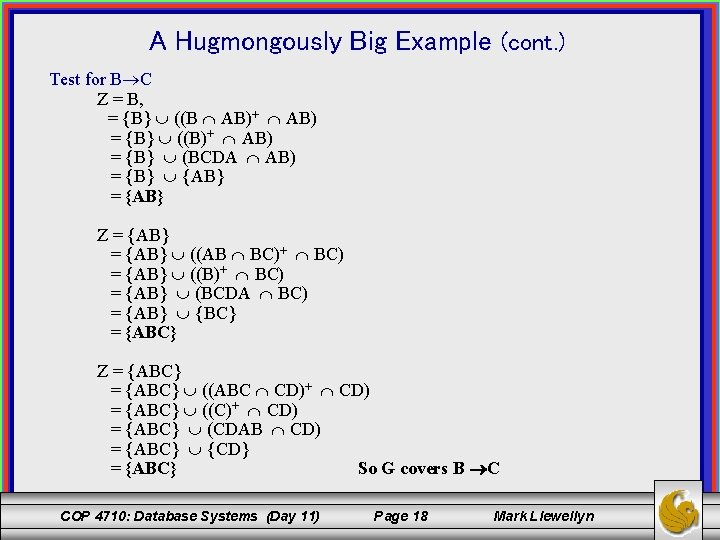A Hugmongously Big Example (cont. ) Test for B C Z = B, = {B} ((B AB)+ AB) = {B} ((B)+ AB) = {B} (BCDA AB) = {B} {AB} = {AB} Z = {AB} ((AB BC)+ BC) = {AB} ((B)+ BC) = {AB} (BCDA BC) = {AB} {BC} = {ABC} Z = {ABC} ((ABC CD)+ CD) = {ABC} ((C)+ CD) = {ABC} (CDAB CD) = {ABC} {CD} = {ABC} So G covers B C COP 4710: Database Systems (Day 11) Page 18 Mark Llewellyn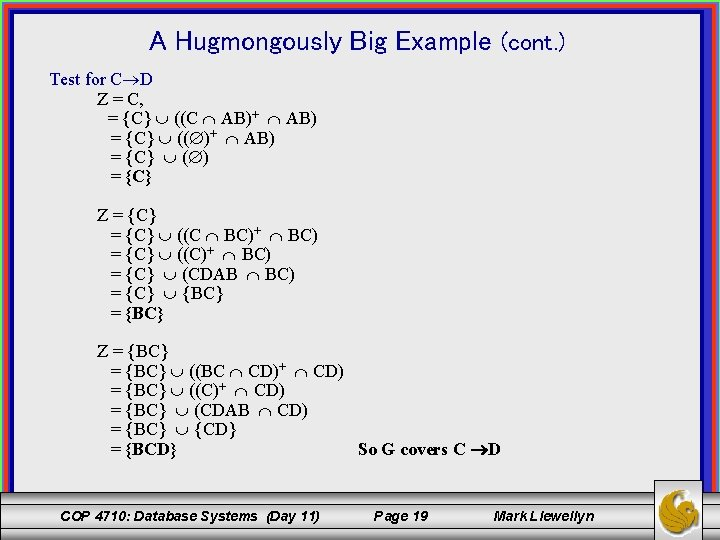A Hugmongously Big Example (cont. ) Test for C D Z = C, = {C} ((C AB)+ AB) = {C} (( )+ AB) = {C} ( ) = {C} Z = {C} ((C BC)+ BC) = {C} ((C)+ BC) = {C} (CDAB BC) = {C} {BC} = {BC} Z = {BC} ((BC CD)+ CD) = {BC} ((C)+ CD) = {BC} (CDAB CD) = {BC} {CD} = {BCD} So G covers C D COP 4710: Database Systems (Day 11) Page 19 Mark Llewellyn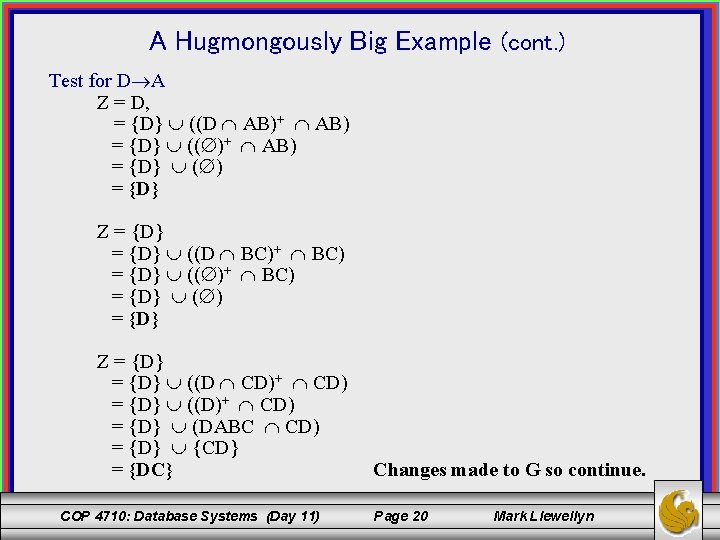A Hugmongously Big Example (cont. ) Test for D A Z = D, = {D} ((D AB)+ AB) = {D} (( )+ AB) = {D} ( ) = {D} Z = {D} ((D BC)+ BC) = {D} (( )+ BC) = {D} ( ) = {D} Z = {D} ((D CD)+ CD) = {D} ((D)+ CD) = {D} (DABC CD) = {D} {CD} = {DC} COP 4710: Database Systems (Day 11) Changes made to G so continue. Page 20 Mark LlewellynA Hugmongously Big Example (cont. ) Test for D A continues on a second pass through D. Z = DC, = {DC} ((DC AB)+ AB) = {DC} (( )+ AB) = {DC} ( ) = {DC} Z = {DC} ((DC BC)+ BC) = {DC} ((C)+ BC) = {D} (CDAB BC) = {D} (BC) = {DBC} Z = {DBC} ((DBC CD)+ CD) = {DBC} ((CD)+ CD) = {DBC} (CDAB CD) = {DBC} {CD} = {DBC} Again changes made to G so continue. COP 4710: Database Systems (Day 11) Page 21 Mark Llewellyn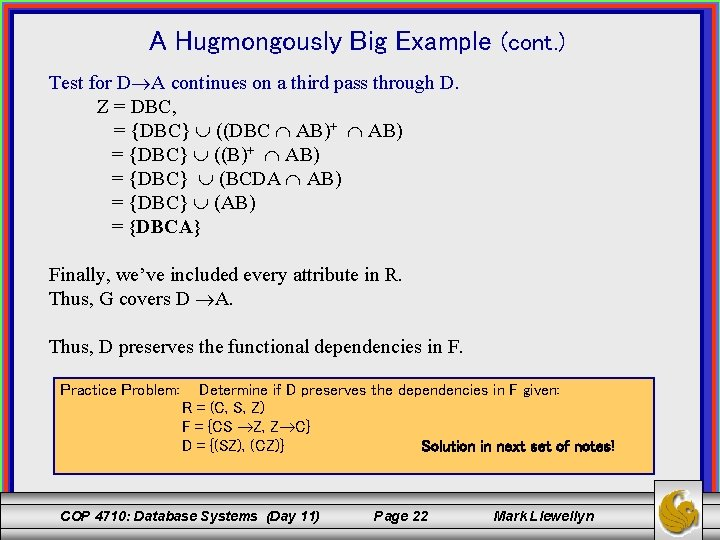A Hugmongously Big Example (cont. ) Test for D A continues on a third pass through D. Z = DBC, = {DBC} ((DBC AB)+ AB) = {DBC} ((B)+ AB) = {DBC} (BCDA AB) = {DBC} (AB) = {DBCA} Finally, we’ve included every attribute in R. Thus, G covers D A. Thus, D preserves the functional dependencies in F. Practice Problem: Determine if D preserves the dependencies in F given: R = (C, S, Z) F = {CS Z, Z C} D = {(SZ), (CZ)} Solution in next set of notes! COP 4710: Database Systems (Day 11) Page 22 Mark LlewellynAlgorithm for Testing for the Lossless Join Property Algorithm Lossless // input: a relation schema R= (A 1, A 2, …, An), a set of fds F, a decomposition // scheme D = {R 1, R 2, . . . , Rk) // output: true if D has the lossless join property, false otherwise Lossless (R, F, D) Create a matrix of n columns and k rows where column y corresponds to attribute Ay (1 y n) and row x corresponds to relation schema Rx (1 x k). Call this matrix T. Fill the matrix according to: in Txy put the symbol ay if Ay is in Rx and the symbol bxy if not. Repeatedly “consider” each fd X Y in F until no more changes can be made to T. Each time an fd is considered, look for rows in T which agree on all of the columns corresponding to the attributes in X. Equate all of the rows which agree in the X value on the Y values according to: If any of the Y symbols is a y make them all ay, if none of them are ay equate them arbitrarily to one of the bxy values. If after making all possible changes to T one of the rows has become a 1 a 2. . . an then return yes, otherwise return no. end. COP 4710: Database Systems (Day 11) Page 23 Mark Llewellyn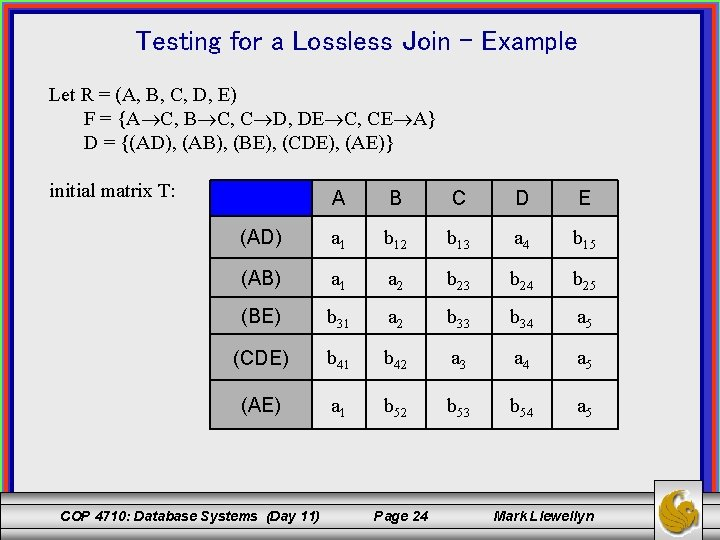Testing for a Lossless Join - Example Let R = (A, B, C, D, E) F = {A C, B C, C D, DE C, CE A} D = {(AD), (AB), (BE), (CDE), (AE)} initial matrix T: A B C D E (AD) a 1 b 12 b 13 a 4 b 15 (AB) a 1 a 2 b 23 b 24 b 25 (BE) b 31 a 2 b 33 b 34 a 5 (CDE) b 41 b 42 a 3 a 4 a 5 (AE) a 1 b 52 b 53 b 54 a 5 COP 4710: Database Systems (Day 11) Page 24 Mark Llewellyn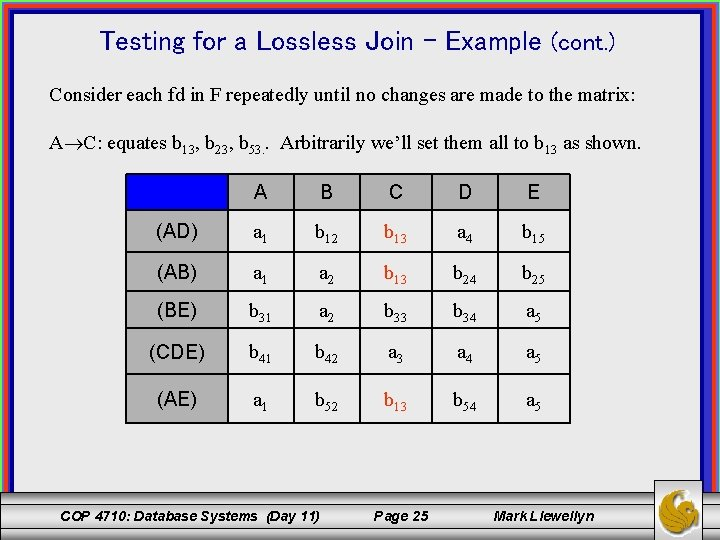Testing for a Lossless Join – Example (cont. ) Consider each fd in F repeatedly until no changes are made to the matrix: A C: equates b 13, b 23, b 53. . Arbitrarily we’ll set them all to b 13 as shown. A B C D E (AD) a 1 b 12 b 13 a 4 b 15 (AB) a 1 a 2 b 13 b 24 b 25 (BE) b 31 a 2 b 33 b 34 a 5 (CDE) b 41 b 42 a 3 a 4 a 5 (AE) a 1 b 52 b 13 b 54 a 5 COP 4710: Database Systems (Day 11) Page 25 Mark Llewellyn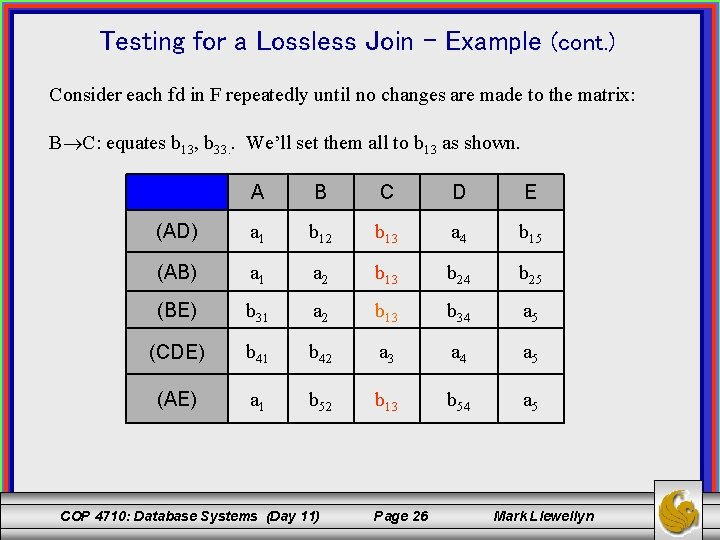Testing for a Lossless Join – Example (cont. ) Consider each fd in F repeatedly until no changes are made to the matrix: B C: equates b 13, b 33. . We’ll set them all to b 13 as shown. A B C D E (AD) a 1 b 12 b 13 a 4 b 15 (AB) a 1 a 2 b 13 b 24 b 25 (BE) b 31 a 2 b 13 b 34 a 5 (CDE) b 41 b 42 a 3 a 4 a 5 (AE) a 1 b 52 b 13 b 54 a 5 COP 4710: Database Systems (Day 11) Page 26 Mark Llewellyn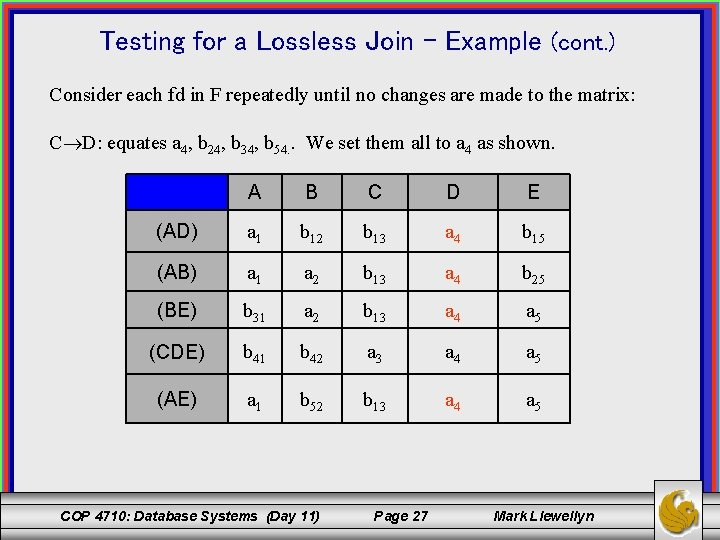Testing for a Lossless Join – Example (cont. ) Consider each fd in F repeatedly until no changes are made to the matrix: C D: equates a 4, b 24, b 34, b 54. . We set them all to a 4 as shown. A B C D E (AD) a 1 b 12 b 13 a 4 b 15 (AB) a 1 a 2 b 13 a 4 b 25 (BE) b 31 a 2 b 13 a 4 a 5 (CDE) b 41 b 42 a 3 a 4 a 5 (AE) a 1 b 52 b 13 a 4 a 5 COP 4710: Database Systems (Day 11) Page 27 Mark LlewellynTesting for a Lossless Join – Example (cont. ) Consider each fd in F repeatedly until no changes are made to the matrix: DE C: equates a 3, b 13. . We set them both to a 3 as shown. A B C D E (AD) a 1 b 12 b 13 a 4 b 15 (AB) a 1 a 2 b 13 a 4 b 25 (BE) b 31 a 2 a 3 a 4 a 5 (CDE) b 41 b 42 a 3 a 4 a 5 (AE) a 1 b 52 a 3 a 4 a 5 COP 4710: Database Systems (Day 11) Page 28 Mark Llewellyn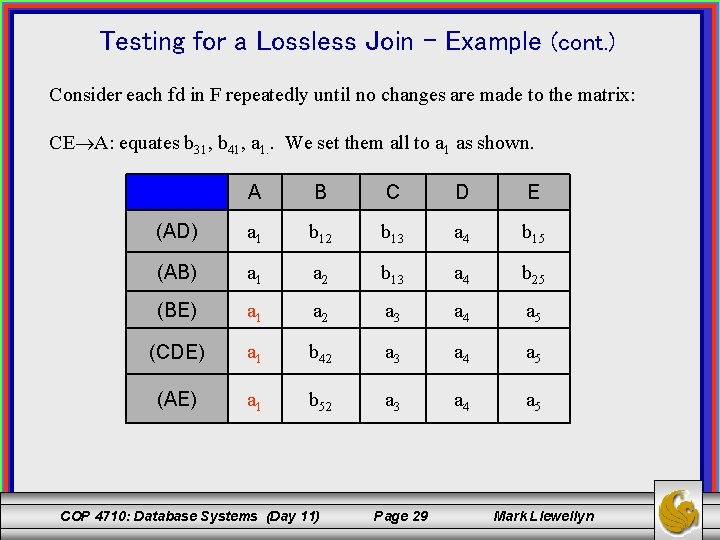Testing for a Lossless Join – Example (cont. ) Consider each fd in F repeatedly until no changes are made to the matrix: CE A: equates b 31, b 41, a 1. . We set them all to a 1 as shown. A B C D E (AD) a 1 b 12 b 13 a 4 b 15 (AB) a 1 a 2 b 13 a 4 b 25 (BE) a 1 a 2 a 3 a 4 a 5 (CDE) a 1 b 42 a 3 a 4 a 5 (AE) a 1 b 52 a 3 a 4 a 5 COP 4710: Database Systems (Day 11) Page 29 Mark Llewellyn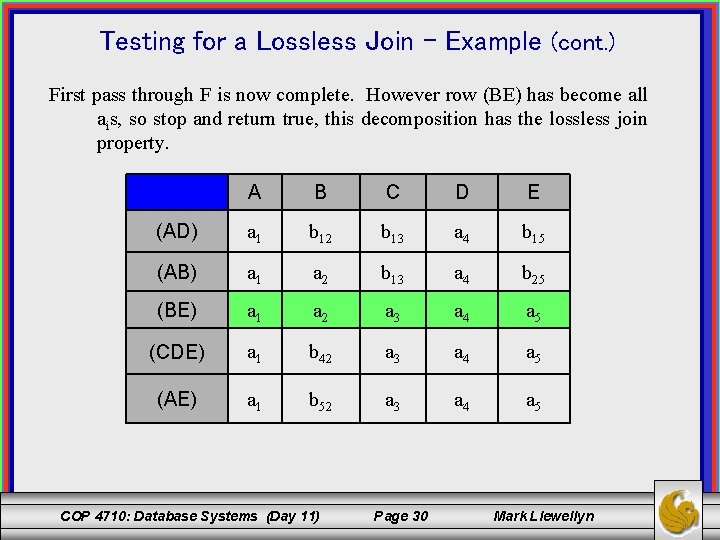Testing for a Lossless Join – Example (cont. ) First pass through F is now complete. However row (BE) has become all ais, so stop and return true, this decomposition has the lossless join property. A B C D E (AD) a 1 b 12 b 13 a 4 b 15 (AB) a 1 a 2 b 13 a 4 b 25 (BE) a 1 a 2 a 3 a 4 a 5 (CDE) a 1 b 42 a 3 a 4 a 5 (AE) a 1 b 52 a 3 a 4 a 5 COP 4710: Database Systems (Day 11) Page 30 Mark Llewellyn